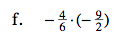### Home > MC2 > Chapter 9 > Lesson 9.3.1 > Problem9-83

9-83.

Simplify each expression. Homework Help ✎

 a.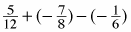b.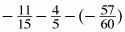c.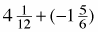d.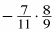e.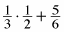f.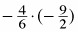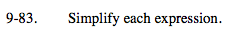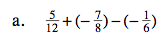Find a common denominator.

$-\frac{7}{24}$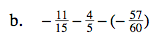Find a common denominator.

$-\frac{44}{60}-\frac{48}{60}-\left(-\frac{57}{60}\right)$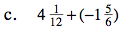Convert these mixed numbers into fractions greater than one.

$\frac{49}{12}-\frac{11}{6}$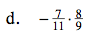$-\frac{56}{99}$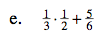$\frac{1}{6}+\frac{5}{6}$